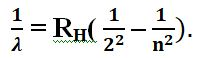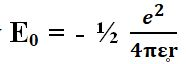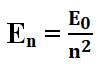# HYDROGEN

20 Mai 2019 by

Hydrogen is the most common element in the Universe. 95% of the atoms in the world are hydrogen, which consists of only one proton spinning around an electron. Seventy-five percent of the Universe’s mass consists of hydrogen, with the rest being helium, which contains two electrons. There are a hundred or so other elements (oxygen, carbon, nitrogen, etc.), which constitute only a few percent of the total. More often than not, hydrogen comes in the form of a very light gas and consequently cannot remain on Earth. In particular, it combines with oxygen to create water, which also makes it very important. Sixty-two percent of our cells consist of hydrogen. It is the main source of energy for the stars, with fusion of it creating helium.

Consequently, what comprises nearly all of our world is the simplest element that nature has ever produced. It is therefore worthy of special attention.

Which is very easy because it is the fundamental atom.

Its electron follows a circular orbit with a radius of 0.5 Angstrom. It releases an energy of 13.6 eV (electron Volts). Its kinetic momentum prompts us to conclude that for a rotation , we get the value ħ, which equals Planck’s constant divided by 2. The proton and the electron are quantum phenomena which are supposed to spin whilst releasing an energy equivalent to ħ/2. It is this fundamental atom which should be considered to constitute most of the Universe. How is it formed? Probably an electron approaches a proton, with the proton and the electron being attracted by the same electrostatic force, the symbol of which is e. This force is inversely proportional to the square of their distance, which means that it extends its influence via spherical layers. But this distance cannot be nil; in other words the two particles cannot be superimposed, because then their energy would be infinite, which obviously has to be ruled out. The result of this is that the electron directs its pulse by rotating as close as possible to the proton. This is the source of the matter we are made of. This arrangement is particularly stable or, in other words, if its movement is modified, it automatically returns to its original configuration. This mechanism is the precondition to the permanence of this fundamental hydrogen atom when it comes to constituting the framework of all of the matter grafted from it, creating a hundred or so sorts of atoms that only comprise approximately 4 % of the mass of the Universe. These atoms combine thanks to a small packet of energy called a “photon”. (See the article entitled “The Black Body”)

Let’s see how this is possible.

The photon is a set of closely grouped waves. This gives it a special characteristic which is its frequency f or the number of beats per second. By multiplying f by Planck’s constant h (there it is again), we obtain the energy of the photon E = hf.

This fundamental atom is capable of swelling, which increases the orbit of its electron to various values, numbered 1, 2, 3…. n. These are meta-stable energy levels of the electron, or in other words, levels that can collapse abruptly to a lower level. A kinetic momentum, , is linked to each level. For each level to have a certain stability, the wave has to be stationary. This means that the path must be such that it is a multiple of the wavelength. The wave is thereby propagated completely in phase with the preceding wave and reinforces it.

The kinetic momentum is the product of the movement quantity times the radius: mv x r. With the wave-corpuscle duality, we see that we have a wavelength λ = h/mv. At the basic level “1”, the circumference travelled by the electron must be 2r or λ, which gives 2r = h/mv or mvr = h/2= ħ. The kinetic momentum mvr does indeed equal ħ. If it is level n then the kinetic momentum of the orbit n is .

This is the starting point for Bohr’s reasoning, who did not know the relation mv. λ = h proposed subsequently by Louis de Broglie, although this is a confirmation of it.          On top of this, Bohr postulated that if, for example an energy hf is required to shift to a higher level, this will be provided by an incident photon. The level achieved may randomly restore this photon. Thus we can say that the hydrogen atom swells or contracts provided that the energy required for this operation is hf. You therefore have to call on Planck’s discovery h twice in order to explain how a hydrogen atom works. This was to be confirmed brilliantly by Balmer’s formula. What is involved in it? The light emitted by hydrogen breaks down into four spectral rays. Balmer attempted, by trial and error, to find the formula providing the wavelength for these rays.

He proposed:RH is a constant and n is a whole number greater than 2.

Based on his hypothesis, Bohr calculated these wavelengths and noticed that they perfectly matched Balmer’s formula. This was an undeniable success, which would not have been possible without his bold hypothesis regarding quantification of the kinetic momentums and the orbits’ jumps in energy levels.

Here are a few more specific details about the hydrogen atom. Its fundamental radius is a0 = 0.5 Angstrom.

Between the proton and the electron, there is a potential negative energy of V.

If the proton is considered to be immobile, the kinetic energy of the electron is T = ½ mv2.

By subtracting T–V you obtain the energywhich has a value of 13.6 electron Volts, and is therefore extremely low. It is the result of the proton and the electron around it coming together.

E0 is a limit value.

There is no detectable energy between E0 and 0.

This is how the fundamental atom is “born”, so to speak. A miracle of nature that can produce this atom with the wave of a magic wand.

But what lies between this atom, which is next to nothing, and nothingness itself? That is a mystery.

At level n, the energy isand the radius of the orbit is an = n2a0

Under the effect of light energy, the atom cannot swell indefinitely. The electrons’ bonds weaken and they end up becoming detached from the atom, and may thereby come to constitute electrical current. What remains is the core, called the “ion”.

The orbits used to be considered circular. But, using more high-performance spectral analysis, it was noticed that the basic rays could disperse into finer rays. Sommerfeld came up with the idea that these orbits may be elliptical.

Associated with each circular orbit of level n, are several elliptical orbits, with each one being characterised by a whole number l, called the “azimuthal quantum number”, such that l > n.

But then it was noted that the energy of the electron does not vary, whether the orbit is circular or elliptical. Sommerfeld thought that this may be attributed to a relativistic effect due to the fact that the velocity of the electron is one hundredth of the speed of light and, in this sense, corrects the electron’s energy levels. The rays obtained are said to be finely structured ones.

The quantum states of the atom are associated with three whole parameters. These are the quantum numbers n, l, and m which correspond to different solutions for the wave function. These states are the consequence of three physical magnitudes: energy, the length of the momentum of the movement quantity or the orbital kinetic momentum, and its projection along a straight line.

n is a principal quantum number and relates to the various energy levels.

l is the orbital quantum number, which can only take the values 0, 1, 2, 3, …, n-1.

m is the magnetic quantum number, which can only take the whole values m = l, l – 1, l – 2, …, 0 – l. It enables the length of the projection passing through the nucleus to be calculated. A moving electron creates an electric current and behaves like a small magnet. A magnetic field is then generated.

In addition to these quantum states, you have to add the spin, which is only found in the quantum world. The choice is limited to two possibilities: rotating clockwise or anti-clockwise.

We have seen that the probabilities of the presence of the electrons were at the maximum level for certain energy layers and sub-layers. An atom’s electrons are spread across these layers, but they can only assume a quantum state at the same time by including the spin state, which gives to opposite possibilities +/- ½ ħ.

This limitation of the electrons’ states is the consequence of their particular spin and the fact that if, within a population of electrons, two of them are permutated, the system’s state vector changes signs. There is therefore a “more or less” choice for each quantum state. The result of this is that the electrons cannot co-exist on the same layer. If there is already one, there can be no room for its opposite. This incompatibility is moreover the key for all matter. It is only built up because electrons, unlike photons, cannot collapse in on themselves. There is an extremely flexible structure, the play of which is determined based on Planck’s constant h. It is thanks to it that matter is constituted. If h was nil, there would be no possibility of reciprocal movements and everything would be fixed and immobile. The possibility of movements and fluctuations is inherent in our world which, without this constant, could not exist. Electrons can move in quite a novel way. Unlike photons for example, they need to make two turns in themselves in order to reattain their original position. “So, for example, unlike photons, they have to make two full rotations to get back to their initial position.”

The electrons’ occupation of the layers can be summarised as follows:

Where n = 1, l = 0, m = 0, there are only two corresponding possibilities, involving spin (  ½).

If n = 2, l equals 0 or 1.

Where l = 0, m = 0 and for l = 1, m it equals 1, 0 or 1.

That gives us 8 possible electrons.

For the nth layer, there can be 2n2 electrons or 18 for layer 3.

In three dimensions, you obtain the following configurations which determine the probability of there being electrons present:

s sphere

p a figure eight

d four-leaf clover shape

f six-leaf clover shape

These are the basic shapes of movements in matter, which determine the structure of atoms and molecules. All this is purely mathematical. There is no evidence that makes it possible to state that these images match reality.

This explanation using geometry enables a visualisation of the phenomena that we can only know via the relations they have between each other.

To round things off, we must stress the structure of the basic atom, which simply features rotational energy which comes on top of a potential energy level.

Bohr presented the atom as being in the shape of a planetary system. But this is only an image. It should not be forgotten that an electron acts in a world where, as we saw, “h” is predominant. In this case, an orbit or a velocity cannot be attributed to the electron. Given the uncertainty relations, neither the position nor the velocity can together have the same levels of accuracy. The trajectory concept has fallen out of favour. While there is no doubt about its individual nature, an electron can only be located within an atom inside a probability cloud. You can simply say that there is a greater chance of finding it there than elsewhere. The orbit is in fact only the location where it is most likely to be. Its shape is only due to the proton’s attraction. Across its orbit, the electron will be more or less likely to be located. This variation in terms of its presence is considered to be a probability wave, but it is not a wave in the physical sense of the term. It is the probability of the electron’s presence that oscillates. This notional wave will travel along the orbital path, with this being circular or elliptical. The presumed wave will therefore create interference with itself. The substantial parts (those where there is a greater presence), will coincide with the hollow parts. What will occur is the same thing that happens when a violin string vibrates; namely there are nodes and anti-nodes. The wave frequency also has to be a function of whole numbers. In the atom, the orbit will become superimposed depending on their presence, with an anti-node for fundamental level 1 and a corresponding number of anti-nodes for the level of the electron. There can be as many as 51 of these in Rydberg’s atom. So it may be said that the atom “resonates” like a circular violin. It is the energy contributed by a photon that enables a shift from one level to another, thereby increasing the number of anti-nodes. This photon reappears if the electron’s energy level falls.

The whole mechanism of the world and its life can be encapsulated in this.

A quite particular phenomenon occurs within the atom. This is the overlapping that you can find in multiple cases in quantum physics. So what is involved? The energy levels are considered to be stationary states in an unstable equilibrium. Shifting from one state to another is done via a sort of third state. It is considered to be “both” the levels to be linked; for example level 1 and level 2. It is difficult to qualify it.

You could perhaps say that the “latent state” holds, in terms of power, the possibility of being level n or level n – 1. This is a state that is “both” in the state of level n and of level n – 1. “Both” can be symbolised exclusively by “And”. And this state is “Neither” level n, “Nor” level n – 1. Instead, it switches from one to the other close level if it comes into contact with the environment. Then it is level nor” level n – 1 which is chosen and becomes temporarily stable. For example, this is the case if you want to know which level is chosen; you destroy the overlapping by observing it. Our logic can only back the assertion that is one extreme “or” its opposite, with the intermediate situation being excluded. However, this is the basis of quantum physics because it characterises the “probable” with a likelihood of occurrence that is the square of the propensity to become established at one of the extremes.

### Tags

Ce site utilise Akismet pour réduire les indésirables. En savoir plus sur comment les données de vos commentaires sont utilisées.

Shares